# QlikView Documents

Documents for QlikView related information.

# How to use - MaxString & MinStringMVP

## How to use - MaxString & MinString

Bullet points:

• MaxString and MinString belongs to Qlikview String Aggregation function.
• They are equivalent to MAX and MIN for integers
• They return the highest and lowest string value

What actually a value of a string?

This is the value described by ANSI character standard where 0 ( NULL) is equal  to 0 and ÿ to 255.

(For full ANSI character set please attached dosument)

Taking only the standard English alphabet (A-z)  ‘A’ will be the MIN and ‘z’ will be the MAX.

Remember:

In ANSI standard A < a and Z < z

(A=65,Z=90,a=97,z=122)

Language specific characters will be not recognized in the place where they are in your alphabet but they will be 'pushed' to the end

(see ANSI table)

MinString and MaxString works on dimensions (columns) only so you cannot use is to evaluate single character or strings .

(MaxString(‘a’,’z’…) is not supported

Examples:

1)    MIN & MAX (textbox)

DataExpressionResultsMinString(Category)

MaxString(Category)2) MIN and MAX of String per group (Straight table)

DataExpressionResult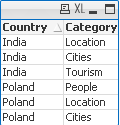MinString(Category)

MaxString(Category)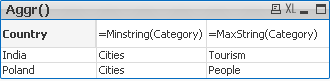3) With condition:

Return Max and Min string based on another column

We are looking for MIN and MAX string value from column Category where Country='Poland'

DataExpressionSolutionResultMinString( if(Country='Poland', Category, null()) )

MaxString( if(Country='Poland', Category, null()) )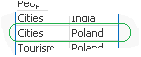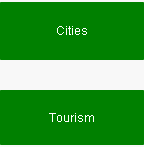4) With LEFT/RIGHT

When used with LEFT the result will be the same as without as the value will be still based on first characters.

DataExpressionSolutionResult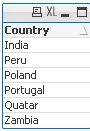=MinString( Right(Country,1))

=MaxString( Right(Country,1))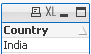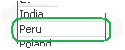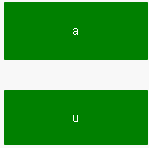5) With MID

DataExpressionSolutionResult=MinString( Mid(Country,2,2))

=MaxString( Mid(Country,2,2))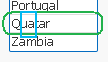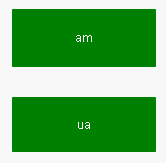6) With Substring

When our data are little scrambled -TextBetween will return string between '.' and end of the row.

DataExpressionResult=MinString(TextBetween(Country,'.','',1))

=MaxString(TextBetween(Country,'.','',1))7) In set analysis

When Criteria of Sales is 2 return Country with highest and lowest string Value

DataExpressionResult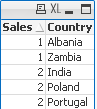=MinString({<Sales={2}>} Country)

=MaxString({<Sales={2}>} Country)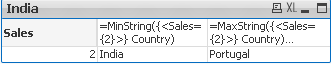😎 With Aggr()

For each Country return lowest and highest Category string value.

DataExpressionResult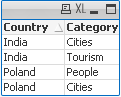=Aggr(Minstring(Category),Country)

=Aggr(Maxstring(Category),Country)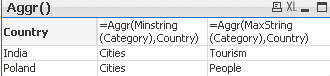If know about other ways of using these functions please let me know.

Labels (8)

• ### New to QlikView

CommentsNot applicable

Hi all,

Please can you kindly explain why number 4 (With LEFT/RIGHT)

is:

=MinString( Right(Country,1))   India

=MaxString( Right(Country,1))  Peru

Instead of:

=MinString( Right(Country,1))   India

=MaxString( Right(Country,1))  ZambiaMVP

=MaxString( Right(Country,1))

Deliver last value from the string

So we havelast values are:

a,u,d,l,r,a

In ANSI standard we have:

a=97

d=100

l=108

r=114

u=117

so MaxString returns 'u'

and MinString='a'Not applicable

Thanks Robert, I understand it now.

Cheers

New Contributor III

Hello!

please tell me how to derive value prior maksstring?

For example, I work expression =MaxString(Month)

but the expression does not work =MaxString(Month-1)MVP

How does your Month data look like?

are the numbers or string characters?

MaxString works on strings only for numbers use Max

New Contributor III

Thanks for the quick response!

expression =MaxString (Month)-1  produces a figure we need to get the name of the month.

New Contributor II

Robert,

Thank you so much for the explanation!

RGS!

Daniel.MVP

I started my QlikView journey. Simply superb.

thanksMVP

So Robert,

Here what is the action running. Can you please explain me

=Replace(MinString({<[Text Boxname] = {'Document\TXout-a-m1-b-t-1'}>} Comment), '|', chr(10))

New Contributor III

Thank Robert,

Very good explanation of functions!Regards,

Joseph

Version history
Revision #:
1 of 1
Last update:
‎2015-03-01 02:48 PM
Updated by: